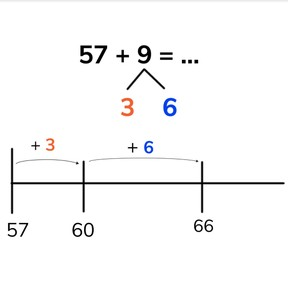8,000 schools use Gynzy92,000 teachers use Gynzy1,600,000 students use Gynzy

## General

Students learn to add to 100 by making ten. They split the second addend into whichever number is required to make ten and then add the rest using the number line.

## Relevance

Discuss with students that you can use adding by tens and ones in addition problems where you can't do the problem in your head right away. An example is if there are 23 kids in class and 9 more come in, you can use adding by tens and ones to calculate that there are 32 kids in total.

## Introduction

Practice decomposing numbers to 10. Students need to be comfortable with this to do calculation with making tens.

## Development

Explain that by making tens you split the second addend into smaller chunks that are easier to add to the first addend. Explain that you set the first addend at the start of the number line and then make ten. Discuss how many it took to make ten and then continue adding on the rest of the numbers. Ask students what the total is. Practice this again. Have students set the first number at the start of the number line, make ten, and then count on with their split second addend.

Check that students understand adding to 100 by making tens regrouping tens by asking the following questions:
- Which number do you jump to first on the number line to make calculation easier?
- What do you need to be able to do to be able to regroup numbers (decompose) numbers.

## Guided practice

Students practice by first making the steps on a number line to make ten and then add on. Next students must take the given jumps and add them to a total, and finally students must determine which steps to take as well as regroup tens.

## Closing

Discuss with students that it is important to be able to add to 100 making tens on the number line because it makes addition easier. Ask students to solve an addition problem shown on the number line. Then have them solve an addition problem on the number line where they need to determine the steps taken themselves. Have students discuss which steps they took on the number line and encourage students to discover that there are many ways to reach the correct total.

## Teaching tips

Students who have difficulty making tens can make use of a number line. Have them draw everything on the number line. Emphasize that they should make ten first, and then continue counting on the number line.

### The online teaching platform for interactive whiteboards and displays in schools

• Save time building lessons

• Manage the classroom more efficiently

• Increase student engagement